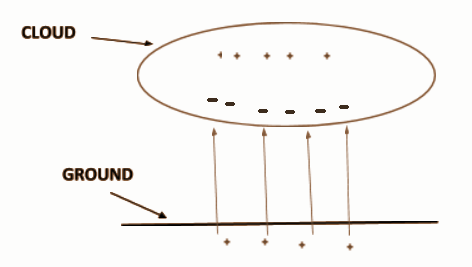# What causes lightning?(A)Excessive electric charge in the atmosphere.(B)Increased chemical energy in the atmosphere.(C)Attraction between two oppositely charged clouds.(D)All of the above.Verified
177.9k+ views
Hint: We know that if a negatively charged surface comes closer to another object, the side of the second object closer to the first surface gets positively charged due to electrostatic induction. Also, there is breakdown voltage where the surface passive film breaks down.

Formulae Used: $\Delta V = Ed$
Where, $\Delta V$ is the potential difference between two parallel conductors, $E$ is the electric field between them and $d$ is the distance between them.

Complete Step By Step Solution
Let us assume a cloud about 1km away from the surface of the earth. The cloud let us say it has positive charges in the upper surface and negative at the bottom surface.
Now, due to induction, the ground becomes positively charged.Now, Let us say the electric breakdown occurs at $3 \times {10^6}V{m^{ - 1}}$
Thus, putting these values in the formula, we get
$\Delta V = {\text{ }}3 \times {10^6} \times {10^3}V$
Calculating this, we get
$\Delta V = {\text{ }}3 \times {10^9}V$
Now,
The electrons start flowing from the cloud to the earth forming funnel which is about $1{\text{ }}to{\text{ }}12m$ in diameter and we call that the ‘step leader’. The step leader moves in about $100mile/s$ . So, it reaches the ground in about $5ms$ (millisecond) talking about $\raise.5ex\hbox{$ \scriptstyle 1 $}\kern-.1em/ \kern-.15em\lower.25ex\hbox{$ \scriptstyle 2 $} {\text{ }}C$ to the earth.
Now,
As the charge flows for about $5ms$ , the current is about $100A$ .
The step leader forms a channel of ionized air. A channel full of ions and full of electrons which is an extremely good conductor of electricity. Now the electrons flow through this channel to the earth very fast creating an enormously high current of about $10000 - 100000{\text{ }}A$ heating the air and creating light (the ions recombine) and we get pressure and we get thunder.
Hence, the correct option is (A).

Note
The values we considered are too near proximity and not the exact one. But the terminology and workflow is precisely correct.